# PHP高级编程-回归原生态-数组排序陷阱

02/16 10:00

### 4.2.3 排序陷阱

<?php// 学生数组$students = array( array('name' => '张三', 'point' => 76), array('name' => '李四', 'point' => 98), array('name' => '小明', 'point' => 95), array('name' => '小红', 'point' => 83), array('name' => '阿布', 'point' => 88),);// 按分数从高到低排序usort($students, function($left,$right) {    if ($left['point'] ==$right['point']) {        return 0;    }    return $left['point'] >$right['point'] ? -1 : 1;});// 输出print_r($students); 上面代码运行后，能得到正确的排序结果。看起来没什么问题，对吧？是的，确实看起来没什么问题。因为这里只有5个学生。但是，假设开发的系统是大型的系统，假设要排序的学生有广东省的全部高考学生，以2017年为例，广东省高考人数大概是75.7万，这时性能又会如何？ 让我们简单来做一个模拟的小实验。用事实结合xhprof性能分析工具得出的数据来说话。 先稍微加以改装，在进行排序的前后加上xhprof的性能分析代码。 // 开始xhprof性能分析xhprof_enable();usort($students, function($left,$right) {    if ($left['point'] ==$right['point']) {        return 0;    }    return $left['point'] >$right['point'] ? -1 : 1;});// 结束xhprof性能分析$xhprof_data = xhprof_disable(); 关于xhprof的使用，网上已经有很多资料说明，这里不再详细展开。最终，我们可以看到这样的性能分析报告：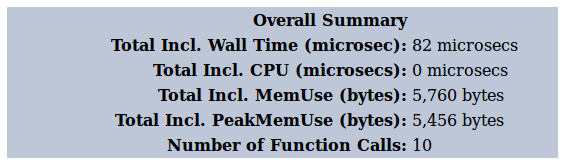图4-1 对5个学生使用usort()排序 接下来，我们可以把学生的数量加大一点。先增加到万的级别，通过指数爆炸很容易做到这一点，以上面5个学生为基础，通过对这5个学生的数据进行11次翻倍后，就可以得到10240组数据。把下面造数据的代码放在$students变量声明后即可。

// 5 * (2 ^ 11) = 10240for ($i = 0;$i < 11;  $i ++) {$students = array_merge($students,$students);}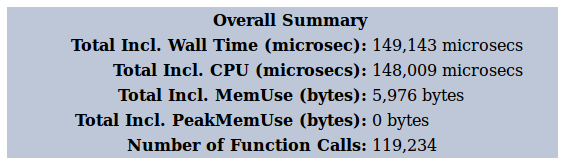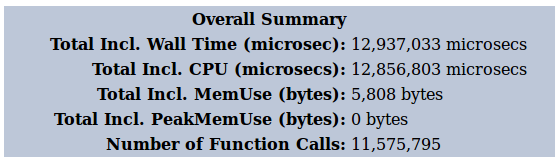5个学生

82毫秒

10次

10,240个学生

149毫秒

119,234次

655,360个学生

11,575,795次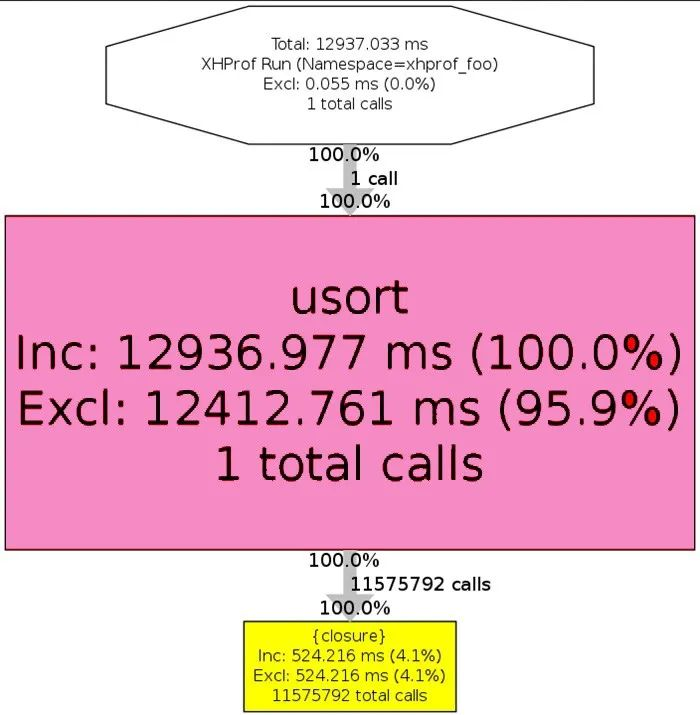// 开始xhprof性能分析xhprof_enable(XHPROF_FLAGS_MEMORY + XHPROF_FLAGS_CPU);$points = array();foreach ($students as $it) {$points[] = $it['point'];} array_multisort($points, SORT_DESC, SORT_NUMERIC, $students);// 结束xhprof性能分析$xhprof_data = xhprof_disable();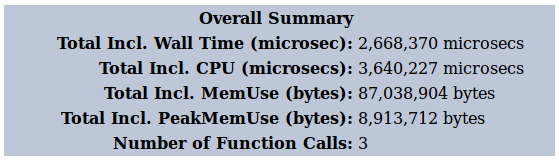<?php// 学生数组$students = array( array('name' => '张三', 'point' => 76), array('name' => '李四', 'point' => 98), array('name' => '小明', 'point' => 95), array('name' => '小红', 'point' => 83), array('name' => '阿布', 'point' => 88),);// 制造更多的学生数据// 5 * (2 ^ 11) = 10240// 5 * (2 ^ 16) = 655360for ($i = 0; $i < 17;$i ++) {    $students = array_merge($students, $students);}// 开始xhprof性能分析xhprof_enable(XHPROF_FLAGS_MEMORY + XHPROF_FLAGS_CPU);//usort($students, function($left,$right) {//    if ($left['point'] ==$right['point']) {//        return 0;//    }////    return $left['point'] >$right['point'] ? -1 : 1;//});$points = array();foreach ($students as $it) {$points[] = $it['point'];} array_multisort($points, SORT_DESC, SORT_NUMERIC, $students);// 结束xhprof性能分析$xhprof_data = xhprof_disable();// print_r($students);$XHPROF_ROOT = realpath(dirname(__FILE__));include_once $XHPROF_ROOT . "/xhprof_lib/utils/xhprof_lib.php";include_once$XHPROF_ROOT . "/xhprof_lib/utils/xhprof_runs.php";// save raw data for this profiler run using default// implementation of iXHProfRuns.$xhprof_runs = new XHProfRuns_Default();// save the run under a namespace "xhprof_foo"$run_id = $xhprof_runs->save_run($xhprof_data, "xhprof_foo");echo "http://localhost/?run=\$run_id&source=xhprof_foo\n";

0
0 收藏

### 作者的其它热门文章0 评论
0 收藏
0